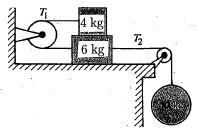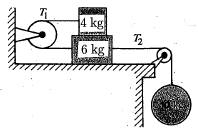# Friction between two stacked objects

PanphobiaThere is friction in between the two stacked objects, but no where else.

Now what I was wondering was when I draw the fbd for the 6 kg square, there is a frictional force in left direction, but is the magnitude of it m1g*coefficient of friction, so (4)(9.8)μ?

Legaldose
This depends on the hanging mass. If they are in equilibrium (not moving) then I would say you are correct. Otherwise you would need to take into consideration the accelerations.

NihalShThere is friction in between the two stacked objects, but no where else.

Now what I was wondering was when I draw the fbd for the 6 kg square, there is a frictional force in left direction, but is the magnitude of it m1g*coefficient of friction, so (4)(9.8)μ?

What is the mass of the hanging ball?

for fbd for 6kg mass, there would be normal forces from floor and mass m1, weight and friction whose direction is towards the left in your situation. the direction is independent of weight of ball. But magnitude depends on it. if its accelerating or on the verge of moving, then you are correct because you have used the condition for max friction. But if the objects are in equilibrium, then friction could range from 0 to max value depending on the mass of hanging weight.

------------------------------------------------------

If they are in equilibrium (not moving) then I would say you are correct. Otherwise you would need to take into consideration the accelerations.
it should depend on the conditions of equilibrium, the answer should be correct only if the block is on the verge of moving. And even considering the acceleration, if there is any, answer should be right given that coefficient of friction doesn't change. Only tension should change for different accelerations. what do you think?

•1 person
Panphobia
I was saying what was weird is that I have to incorporate friction twice, once on the top object and once on the bottom object, and this is kinetic friction "Legaldose", not static friction. Also the system IS accelerating to the right or down.

NihalSh
I was saying what was weird is that I have to incorporate friction twice, once on the top object and once on the bottom object, and this is kinetic friction "Legaldose", not static friction. Also the system IS accelerating to the right or down.

it is not at all weird....it is a consequence of Newton's third law

Panphobia
so the magnitude of this friction is the exact same in both cases correct?

NihalSh
so the magnitude of this friction is the exact same in both cases correct?yes, exactly

Panphobia
I KNEW IT :D, just wanted to make sure I was doing it right :)

NihalSh
I KNEW IT :D, just wanted to make sure I was doing it right :)

great then, glad I could help!!!